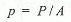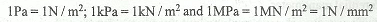# Pressure of a Liquid

When a liquid is contained in a vessel, it exerts force at all points on the sides and bottom of the vessel. The force per unit area is called intensity of pressure. Mathematically, intensity of pressure,where

P = Force acting on the liquid, and
A = Area on which the force acts.

The intensity of pressure at any point, in a liquid at rest is equal to the product of weight density of the liquid (w) and the vertical height from the free surface of the liquid (h) (it is also known as static head). Mathematically, intensity of pressure,

p = w.h

From this expression, we see that the intensity of pressure at any point, in a liquid, is directly proportional to depth of liquid from the surface.

The intensity of pressure may be expressed either in N / m2, N / mm2 or in metres of liquid or mm of liquid. It is also expressed in pascal (briefly written as Pa), such that